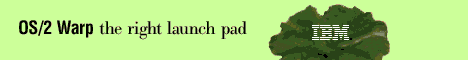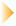the REXX Files - by Dr. Dirk TerrellSorting a list of numbers is a subject that has been studied extensively over the years. There are many different algorithms that have been developed, but none of them are always better than any of the others. Since sorting is a common task, I thought we'd look at some sorting algorithms implemented in REXX. In this article we'll look what is probably the simplest algorithm, the bubble sort, and another one that is a bit more efficient called the straight insertion method. In later articles, we'll look at some other algorithms that are generally much more efficient.

The first thing we need to take care of is generating some random numbers to be sorted. The REXX function RANDOM() generates (pseudo) random numbers. The calling form is

`     random_number=RANDOM(start,end,seed) `
where start and end are the beginning and end of the range of possible random numbers, and seed is a seed value for the random number generator. RANDOM(1,10) would return a random number between 1 and 10. For a given seed value, the same sequence of random number is returned when RANDOM is called with the seed the first time and without a seed value thereafter. All of the parameters are optional. If you specify a single number, the function returns a random number between 0 and that number. If you specify no numbers, the default range of 0 to 999 is used. (Note than this function returns whole numbers. If you need random floating-point numbers, you will have to divide the result by an appropriate value.)

Let's have our program take a number on the command line that tells the program how many numbers we want to sort. And let's prompt the user for that value if it isn't entered. Here is the code to do this:

```/* Sorting Program */
Parse Arg N
If N="" then Do /* User didn't enter the number of items to sort */
Say "How many numbers to sort?"
Parse Pull N
end /* do */

/* Generate some random numbers */
Do i=1 to N
x.i=Random(0,N) /* Generate random numbers and save in a stem variable */
x2.i=x.i        /* Save a copy of the random numbers for the later sorts */
end /* do */
x.0=N              /* Set the 0 index equal to the number of items */```
Now that we have a set of random numbers, we are ready to sort them. The bubble sort is a very simple sorting algorithm, but it is not very efficient. For N items, it typically requires of order NxN comparisons. For small N, that is not too bad, but as N grows large, the algorithm becomes impractical as you can see by experimenting with the program we are writing.

The bubble sort works by looping through the list of items, comparing successive pairs. If they are out of order, they are reversed. The loop is repeated until no exchanges have been made, meaning that the list is sorted. The algorithm is therefore very simple, but the price of that simplicity is very poor efficiency as you will see. Here is the code that performs the bubble sort, written as a subroutine that can be called from within the main program:

```BubbleSort:
Procedure Expose x.
start=Time("R") /* Start the timer */
do i=x.0 to 1 by -1 until sorted=1
sorted=1 /* Assume the items are sorted */
do j=2 to i
m =j-1
if x.m>x.j then /* If the items are out of order, swap them */
do
a=x.m
x.m=x.j
x.j=x
sorted=0  /* We swapped two items, so we're not sorted yet */
end /* do */
end /* do */
end /* do */
end=time("R") /* Stop the timer */
elapsed=end-start
Return elapsed /* Return the elapsed time */```
This routine implements the bubble sort algorithm, but also adds the nice touch of returning the elapsed time during the sorting for comparison to other algorithms. One thing about the routine that deserves some explanation is the use of the PROCEDURE statement. Use this when you want the variables of the routine to be hidden from the rest of the program. You can allow other parts of the program to see specific variables, though, by using the EXPOSE statement as we do here with the variable x., the stem that contains the items to be sorted. To sort the items, we just call the bubble sort routine like this:
`     elapsed_time=BubbleSort()`
The straight insertion algorithm is a bit more efficient than the bubble sort algorithm. This algorithm is frequently used by card players to sort a deck. You start with the second card and compare it to the first. Then you take the third card and compare it to the first two, then the fourth compared to the first three, and so on. Here is the REXX code to perform a straight insertion sort:
```InsertionSort:
Procedure Expose x.
start=Time("R")
Do i=1 to x.0-1
Do j=i+1 to x.0
If x.j<x.i then  Do
a=x.i
x.i=x.j
x.j=a
end /* do */
end /* do */
end /* do */
end=time("R")
elapsed=end-start
Return elapsed```
Finally, let's look at a slight variant of the straight insertion. This method works by finding the smallest item in the list and moving it to the front each time through the main loop. This one is, like the others, fairly simple, but in most cases a little more efficient, usually several times faster than the bubble sort. Here is the code:
```ModifiedInsertionSort:
start=Time("R")
Do i=1 to x.0
m=x.i
k=i
do j=i+1 to x.0
if x.j<m then do /* Find the smallest number and */
m=x.j         /* store the value and the index.  */
k=j
end
end /* do */
a=x.i   /* Move the smallest item to the front */
x.i=m
x.k=a
end /* do */
end=time("R")
elapsed=end-start
Return elapsed```
These routines are not bad for small numbers of items. They are very simple and easy to code up compared to some more sophisticated algorithms. For large numbers of items, though, their performance is usually quite poor because they all require of order NxN comparisons (so-called N-squared algorithms). Other algorithms scale better with increasing numbers of items, but we pay for improved efficiency with more complicated code.

We'll look some of these more sophisticated techniques next time. Until then, have fun playing around with the sample code and seeing if you can come up with more efficient sorting algorithms.Dr. Dirk Terrell is an astronomer at the University of Florida specializing in interacting binary stars. His hobbies include cave diving, martial arts, painting and writing OS/2 software such as HTML Wizard.

[Our Sponsor: Perez Computing Services - Makers of Ctrl-Alt-Del Commander and IPF Editor.]